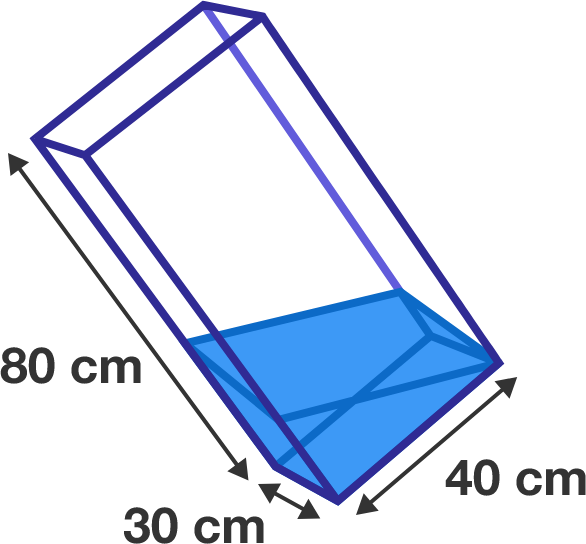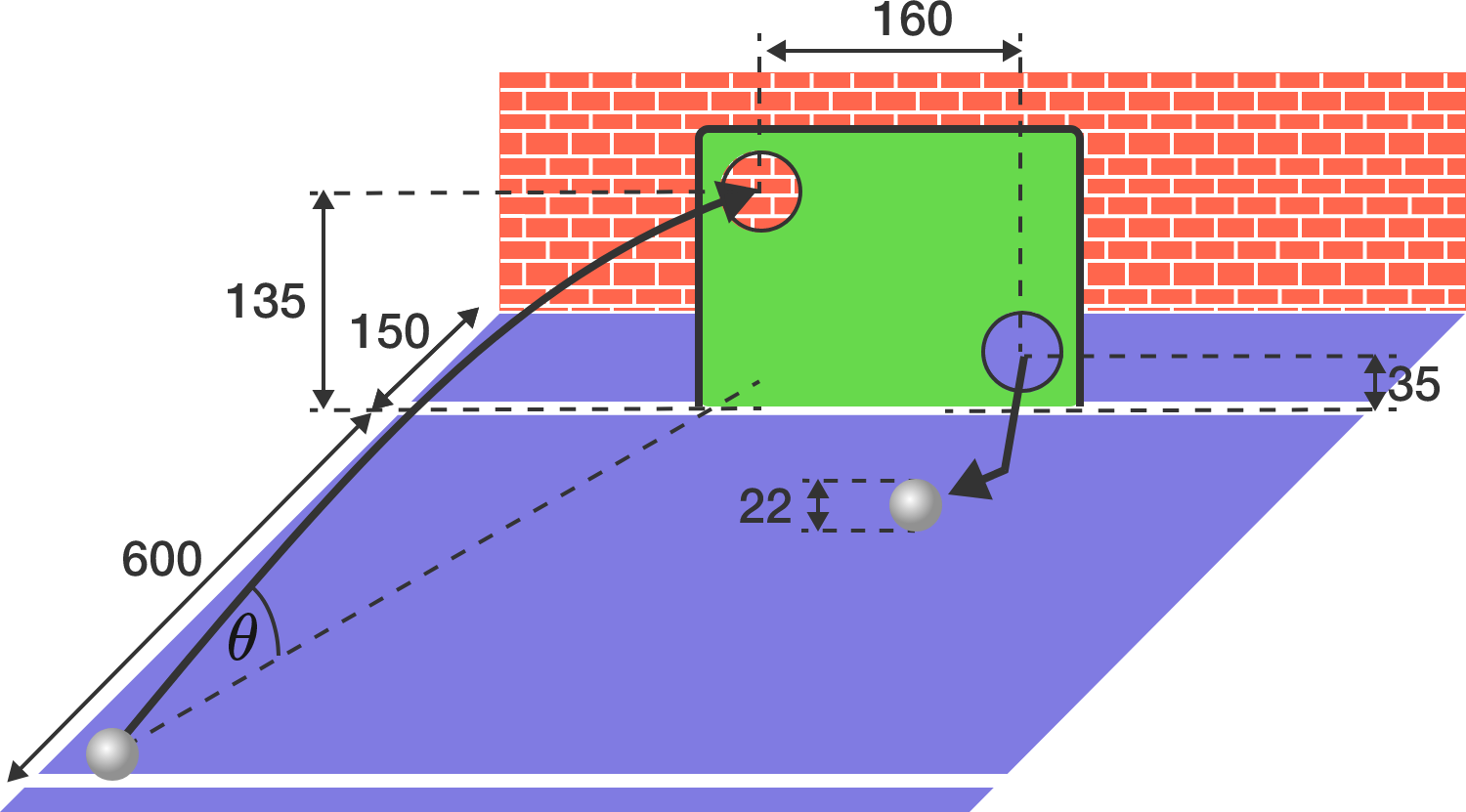# Problems of the Week

Contribute a problem

What can we say about positive real solutions to the equations

$\Large x^{{x}^{2018}} = 2018\quad \text{ and }\quad x^{x^{x^{^ {.^{.^.} } } }} = 2018\,?$

Is it possible to color every positive real number so that

• each number is colored one of 10 distinct colors, and
• any two numbers that differ in exactly one digit are colored differently?

For example, the ten numbers ${\color{maroon}0.111} \ldots,\, {\color{#D61F06}1.111}\ldots,\, {\color{#EC7300}2.111} \ldots,\, {\color{limegreen}3.111} \ldots,\, {\color{#20A900}4.111} \ldots,\, {\color{teal}5.111} \ldots,\, {\color{#3D99F6}6.111} \ldots,\, {\color{#0C6AC7}7.111} \ldots,\, {\color{#302B94}8.111} \ldots,\, {\color{magenta}9.111} \ldots$ must all be different colors because any two of them differ in exactly one digit. However, the numbers $10.000 \ldots$ and $1.000 \ldots$ do not have to be colored differently because they differ in more than one digit.

You may assume the axiom of choice holds.A water tank is in the shape of a cuboid with a height of $80\text{ cm}$ and a base that measures $30\text{ cm} \times 40\text{ cm}.$

It is tilted so that its space diagonal (the longest diagonal between two opposing vertices) becomes vertical.

Next, water is poured into the tank up to the highest point of the tilted base, as shown in the diagram to the right.

How much water (in cubic centimeters) has been poured in?

$A$ is a non-empty proper subset of $\mathbb Z$ with at least two elements, and with the following property:

If $a$ and $b$ are distinct elements of $A,$ then $-a,$ $a+2b,$ and $a+19$ are also elements of $A.$

Find $\min \limits_{a \in A \atop a \neq 0}\big(|a|\big).$

Billy exercises shots on a goal wall to improve his soccer skills. The goal wall has two holes that serve as targets for the ball with diameter $d = 22\, \text{cm}$. Since he already can hit both holes from a short distance relatively reliably, he is looking for a new challenge. Billy considers a trick shot in which he hits both holes with a single shot. He uses the fact that directly behind the goal wall is a brick wall, where the ball can bounce off. If he hits the hole at top left with a diagonal shot, then it is possible that the ball rebounds on the back wall and then reaches the second hole at bottom right. But what should the optimal shooting angle $\theta$ be? As a result, enter the numerical value for $\tan \theta$ (the initial slope of the trajectory) with an accuracy of two decimal places.Details and Assumptions: Billy shoots at the goal from the white line, which is 6 meters apart from the goal line. Behind the goal wall is the brick wall at a distance of 1.5 meters. The two holes in the goal wall have a horizontal distance--with respect to their centers--of 1.6 meters, and a height of 1.35 and 0.35 meters above the ground, respectively. (All geometrical dimensions are also drawn in the diagram in units of centimeters.) The holes are sufficiently large to allow the ball to pass through. For the optimal shot, the ball should pass through the centers of the holes.

We assume that the ball does not rotate around its own axis and rebounds completely elastically on the back wall. The back wall is also completely flat and even (the brick pattern is in reality just a wallpaper). When the ball hits the back wall, it deforms to a hemisphere. We can therefore treat it as a mass point located at the center of the ball during this bounce. Although the ball must first be accelerated by Billy's foot to reach its initial speed, we assume that this acceleration phase is only negligibly short. Thus, the ball starts directly on the ground with a certain initial velocity and is subject only to the gravitational force (frictional forces are therefore not considered).

×